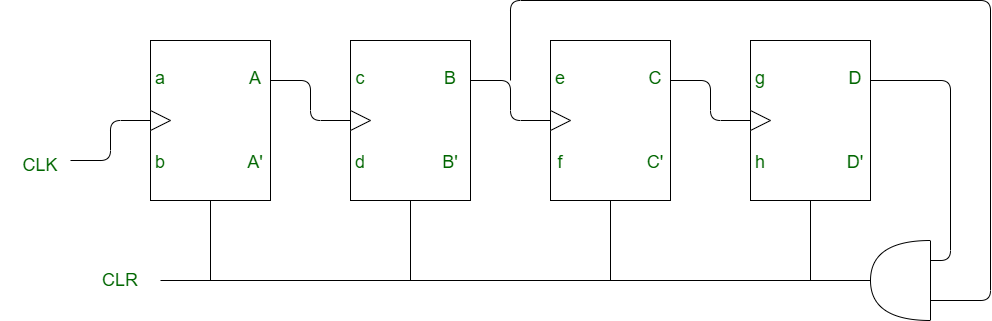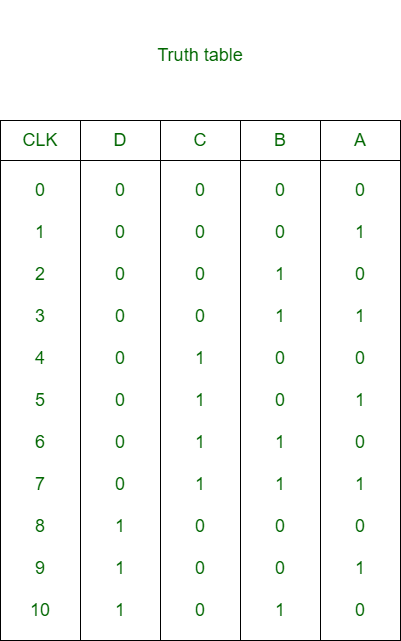# Non-binary Counter in Digital Logic

A counter is a circuit that counts the number of occurrences of an input. The circuit consists of flip-flops which along with combinational elements are used for generation of control signals.

```If M = Total number of states, and
n = Total number of flip-flop
Then,      M <= 2n
If         M = 2n    ; Binary counter
and        M < 2n    ; Non-binary counter ```

Block Diagram of Non-Binary Counter:
Here is the block diagram of a mod-10 counter. Here, the total number of flip-flops required is 4 thus the number of used state is 10 and number of unused state is 6.In order to design a non binary counter a logic gate is required which detects M stage. In mod-10 coounter 10 stages are detected from 0000 to 10001 and as soon as 1010 appears it clears all the flip-flops.

Making of Non-binary counter:
For making non-binary counter,

• If CLR is present and CLK is connected with output Q, then we use AND gate.
• If CLR is present and CLK is connected with output Q’, then we use NOR gate.
• If complement of CLR is present and CLK is connected with output Q, then we use NAND gate.
• If complement of CLR is present and CLK is connected with output Q’, then we use OR gate.

Truth Table:It is clear from truth table that as soon as 1010 appears, inputs to the AND gate becomes 11 which resets the flip-flop with CLR =1.

Output Frequency:
The output frequency of mod-M counter,

`= f / M `

If there is no feedback present at last output (i.e D) then the output frequency,

`= f / (2n) `

Don’t stop now and take your learning to the next level. Learn all the important concepts of Data Structures and Algorithms with the help of the most trusted course: DSA Self Paced. Become industry ready at a student-friendly price.

My Personal Notes arrow_drop_upCheck out this Author's contributed articles.

If you like GeeksforGeeks and would like to contribute, you can also write an article using contribute.geeksforgeeks.org or mail your article to contribute@geeksforgeeks.org. See your article appearing on the GeeksforGeeks main page and help other Geeks.

Please Improve this article if you find anything incorrect by clicking on the "Improve Article" button below.

Article Tags :

Be the First to upvote.

Please write to us at contribute@geeksforgeeks.org to report any issue with the above content.